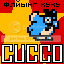-=+=- -=+=- -=+=- -=+=- -=+=- -=+=- -=+=- -=+=- -=+=- -=+=- -=+=- -=+=- -=+=- -=+=- -=+=- -=+=- -=+=- -=+=- -=+=- -=+=- -=+=- -=+=- -=+=- -=+=- -=+=- -=+=- -=+=- -=+=- -=+=- -=+=- (c) WidthPadding Industries 1987 0|91|0 -=+=- -=+=- -=+=- -=+=- -=+=- -=+=- -=+=- -=+=- -=+=- -=+=- -=+=- -=+=- -=+=- -=+=- -=+=- -=+=- -=+=- -=+=- -=+=- -=+=- -=+=- -=+=- -=+=- -=+=- -=+=- -=+=- -=+=- -=+=- -=+=- -=+=- SoCoder -> Link Home -> Downloadable Games Great Freeware/Shareware/Abandonware games. Older --> Cloud Game Dan , WindowsCommander Keen... Mods! HoboBenSonic Fan Remix JayenkaiCommander Keen 6 and 3.5 HoboBenCommander Keen beta discovered on 20 year old disk! HoboBenThe Ur-Quan Masters An open-source remake of Star Control 2 HoboBenCave Story Classic side-scrolling adventure PhoenixZeno Clash - £3.82 HoboBenPay £2.50+ for Championship Manager JayenkaiTES2: Daggerfall Bethesda are giving away this classic game to celebrate 15 years of The Elder Scrolls flying_cuccoBeautiful indie game HoboBenN.I. Windows Games 100 100 games for windows in 15MB (shareware) PioMount and Blade Demo HoboBenLOL... steve_ancellCommander Keen - Episodes 1 to 5 - 50% off! HoboBenSinksub HoboBenPhantasy Star Online running on a free server JL235 Dwarf Fortress LostMember Pocket Tanks Best scorched remake ever. LostMemberMight And Magic tribute An unofficial M&M tribute site HoboBenJet Set Willy : Online Multiplayer Willy! JayenkaiAvernum RPGs Great RPG demos that work on Windows and the Mac. HoboBenTCKSOFT Freeware Remakes caffeinekid Older -->